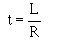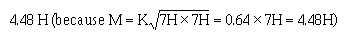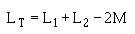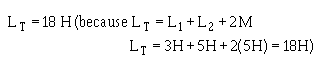Inductance is the property of a coil (or circuit) which opposes any CHANGE in current. A4. Induced emf is the emf which appears across a conductor when there is relative motion between the conductor and a magnetic field; counter emf is the emf induced in a conductor that opposes the applied voltage. A5. The induced emf in any circuit is in a direction to oppose the effect that produced it. A6. No effect. ">Custom SearchANSWERS TO QUESTIONS Q1. THROUGH Q17. A1. The henry, H. A2. Magnetic field. A3. Inductance is the property of a coil (or circuit) which opposes any CHANGE in current. A4. Induced emf is the emf which appears across a conductor when there is relative motion between the conductor and a magnetic field; counter emf is the emf induced in a conductor that opposes the applied voltage. A5. The induced emf in any circuit is in a direction to oppose the effect that produced it. A6. No effect.     Inductance opposes any change in the amplitude of current. A7. The numbers of turns in a coil.     The type of material used in the core.     The diameter of the coil.     The coil length.     The number of layers of windings in the coil.     Increases inductance.     Increases inductance.     Decreases inductance.     Increases inductance.     Increases inductance. A8. Inductance causes a very large opposition to the flow of current when voltage is first applied to an LR circuit; resistance causes comparatively little opposition to current at that time. Zero. During current buildup, the voltage across the resistor gradually increases to the same voltage as the source voltage; and during current decay the voltage across the resistor gradually drops to zero. A9.A10. 1.71 amperes.      5 time constants.      2 time constants. A11. Copper loss; hysteresis loss; eddy-current loss. A12. Mutual inductance is the property existing between two coils so positioned that flux from one coil cuts the windings of the other coil. A13. When they are arranged so that energy from one circuit is transferred to the other circuit. A14. The ratio of the fines of force produced by one coil to the lines of force that link another coil. It is never greater than one. A15.A16.A17.Integrated Publishing, Inc. - A (SDVOSB) Service Disabled Veteran Owned Small Business